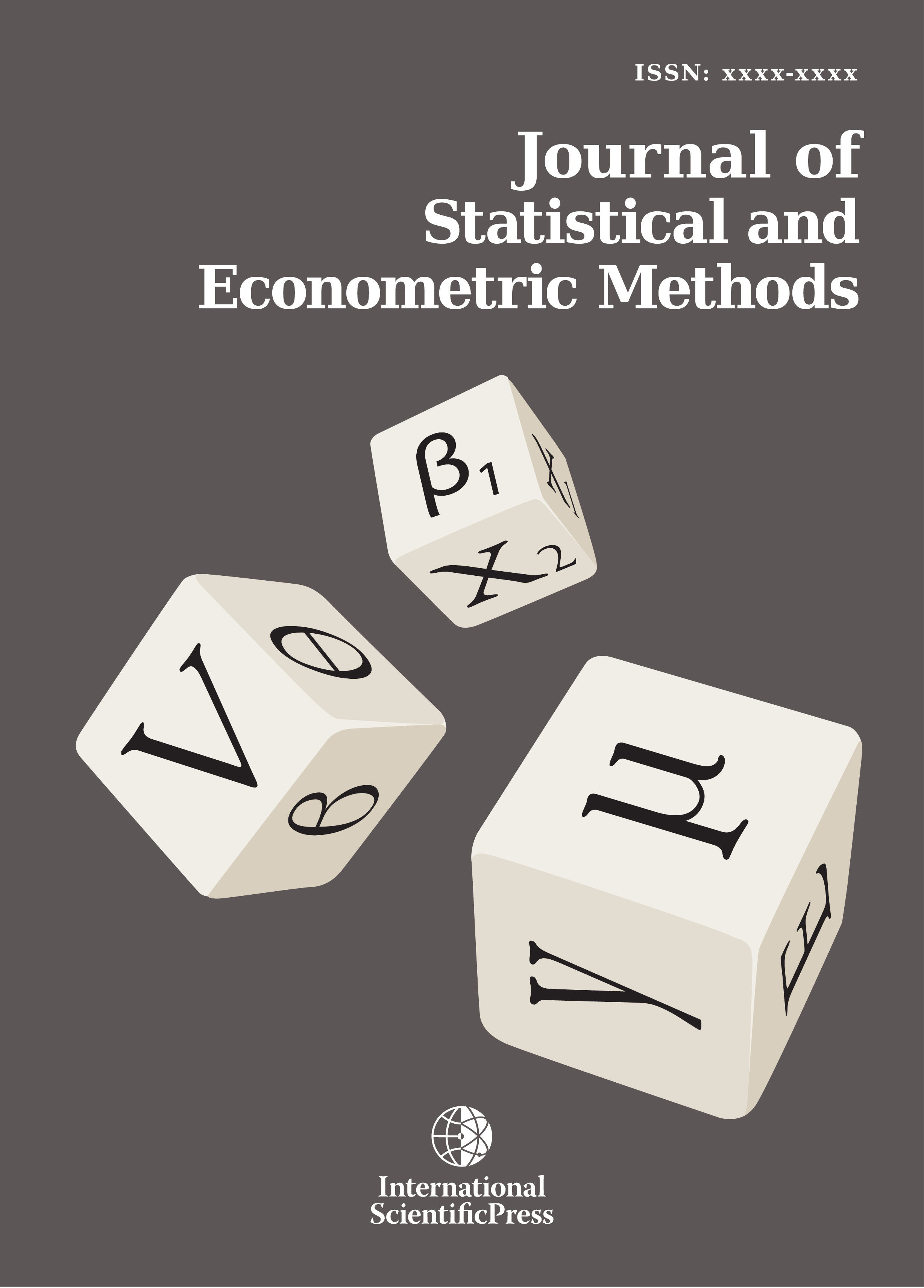# Journal of Statistical and Econometric Methods

#### T-statistic for Autoregressive process

•[ Download ]
• Times downloaded: 152
• Abstract

In this paper, we discuss the distribution of the t-statistic under the assumption of normal autoregressive distribution for the underlying discrete time process. This result generalizes the classical result of the traditional t-distribution where the underlying discrete time process follows an uncorrelated normal distribution. However, for AR(1), the underlying process is correlated. All traditional results break down and the resulting t-statistic is a new distribution that converges asymptotically to a normal. We give an explicit formula for this new distribution obtained as the ratio of two dependent distribution (a normal and the distribution of the norm of another independent normal distribution). We also provide a modified statistic that is follows a non central t-distribution. Its derivation comes from finding an orthogonal basis for the the initial circulant Toeplitz covariance matrix. Our findings are consistent with the asymptotic distribution for the t-statistic derived for the asympotic case of large number of observations or zero correlation. This exact finding of this distribution has applications in multiple fields and in particular provides a way to derive the exact distribution of the Sharpe ratio under normal AR(1) assumptions.

Mathematics Subject Classification: 62E10; 62E15
Keywords: t-Student; Auto regressive process; Toeplitz matrix; circulant matrix; non centered Student distributionISSN: 2241-0376 (Online)
2241-0384 (Print)